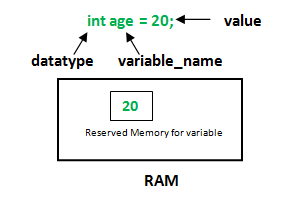# C++ 变量类型

C++ 中每个变量都有指定的类型，类型决定了变量存储的大小和布局，该范围内的值都可以存储在内存中，运算符可应用于变量上。我们可以把变量理解为程序可操作的某个存储区域的别名。## C++ 中的变量定义

C++ 中变量定义的语法如下：

type variable_list;


• type 必须是一个有效的 C++ 数据类型，比如 char、wchar_t、int、float、double、bool 或其他任何用户自定义的对象；
• variable_list 可以由一个或多个标识符名称组成，多个标识符之间用逗号分隔。

int    i, j, k;
char   c, ch;
float  f, salary;
double d;


type variable_name = value;


extern int d = 3, f = 5;    // d 和 f 的声明
int d = 3, f = 5;           // 定义并初始化 d 和 f
byte z = 22;                // 定义并初始化 z
char x = 'x';               // 变量 x 的值为 'x'


## C++ 中的变量声明

#include <iostream>
using namespace std;

//变量声明
extern int a, b, c;
extern float f;

int main ()
{
// 变量定义
int a, b, c;
float f;

// 实际初始化
a = 10;
b = 20;
c = a + b;

cout << c << endl ;

f = 20.0/3.0;
cout << f << endl ;

return 0;
}


\$ ./a.out
30
6.66667


## 左值和右值

C++ 中有两种类型的表达式：

• 左值（lvalue）表达式： 指向内存位置的表达式被称为左值表达式。左值可以出现在赋值号的左边，也可以出现在赋值号的右边。
• 右值（rvalue）表达式： 指的是存储在内存中某些地址的数值。右值是不能对其进行赋值的表达式，也就是说，右值可以出现在赋值号的右边，但不能出现在赋值号的左边。

#define VALUE  10
int val;
val = 20;
val = VALUE;


#define VALUE  10
int val;
VALUE = val;
VALUE = 20;